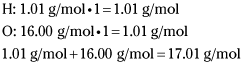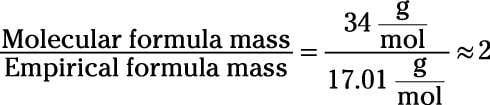##### Chemistry Workbook For Dummies with Online PracticeMany compounds in nature are composed of atoms that occur in numbers that are multiples of their empirical formula. In other words, their empirical formulas don't reflect the actual numbers of atoms within them; instead, they reflect only the ratios of those atoms. What a pain!

Fortunately, this is an old pain, so chemists have devised a means to deal with it. To account for these annoying types of compounds, chemists are careful to differentiate between an empirical formula and a molecular formula. A molecular formula uses subscripts that report the actual number of each type of atom in a molecule of the compound (a formula unit accomplishes the same thing for ionic compounds).

Molecular formulas are associated with gram molecular masses that are simple whole-number multiples of the corresponding empirical formula mass. For example, a molecule with the empirical formula CH2O has an empirical formula mass of about 30 g/mol (12 for the carbon + 2 for the two hydrogens + 16 for the oxygen). The molecule may have a molecular formula of CH2O, C2H4O2, C3H6O3, or the like. As a result, the compound may have a gram molecular mass of 30 g/mol, 60 g/mol, 90 g/mol, or another multiple of 30 g/mol.

You can't calculate a molecular formula based on percent composition alone. If you attempt to do so, the famous chemists (who named the amount of particles in a mole) Avogadro and Perrin will rise from their graves, find you, and slap you 6.02 x 1023 times per cheek. You can clearly see the folly of such an approach by comparing formaldehyde with glucose. The two compounds have the same empirical formula, CH2O, but different molecular formulas, CH2O and C6H12O6, respectively.

Glucose is a simple sugar, the one made by photosynthesis and the one broken down during cellular respiration. You can dissolve it in your coffee with pleasant results. Formaldehyde is a carcinogenic component of smog. Solutions of formaldehyde have historically been used to embalm dead bodies. (Dissolving formaldehyde in your coffee is not advised.) In other words, molecular formulas differ from empirical formulas, and the difference is important in the real world.

## Determining a molecular formula

To determine a molecular formula, you must know the gram formula mass of the compound as well as the empirical formula (or enough information to calculate it yourself from the percent composition). With these tools in hand, calculating the molecular formula involves three steps:
1. Calculate the empirical formula mass.

2. Divide the gram molecular mass by the empirical formula mass.

3. Multiply each of the subscripts within the empirical formula by the number calculated in Step 2.

Here's an example: What is the molecular formula of a compound that has a gram molecular mass of 34 g/mol and the empirical formula HO?
1. Calculate the empirical formula mass.

You determine this number by finding the mass of HO (1 hydrogen atom and 1 oxygen atom).So, the empirical formula mass is 17.01 g/mol.

2. Divide the gram molecular mass by the empirical formula mass.

Dividing the gram molecular mass by this value yields the following:3. Multiply each of the subscripts within the empirical formula by the number calculated in Step 2.

Multiplying the subscripts within the empirical formula by this number gives you the molecular formula H2O2. This formula corresponds to the compound hydrogen peroxide.